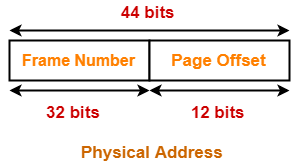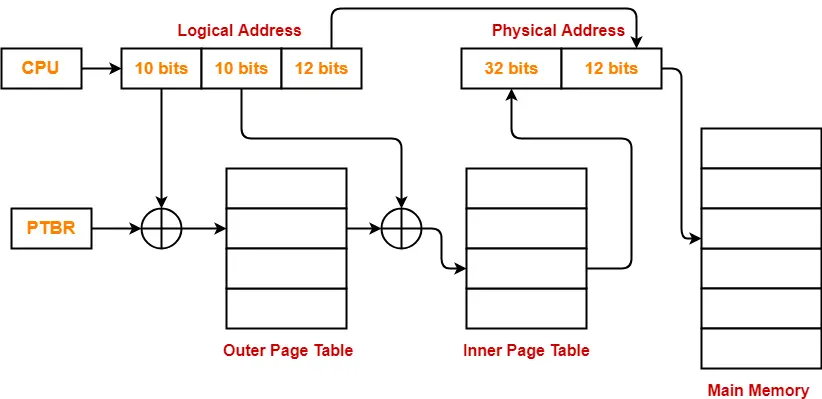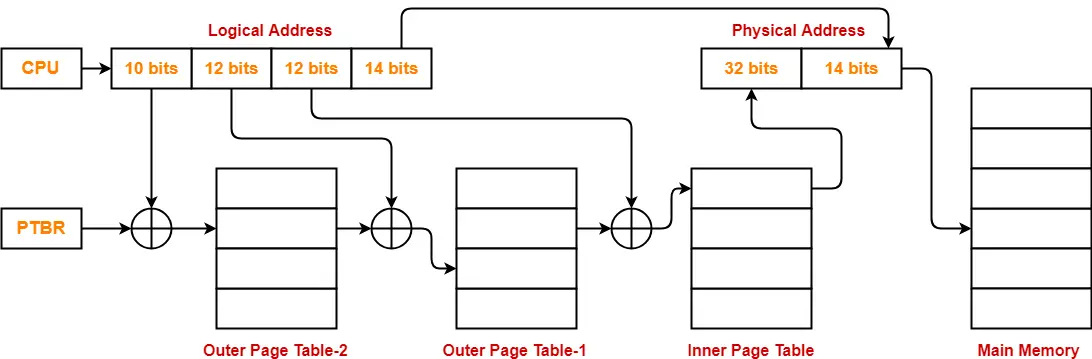# Multilevel Paging in OS | Paging in OS

## Paging in OS-

Before you go through this article, make sure that you have gone through the previous article on Paging in OS.

We have discussed-

• Paging is a non-contiguous memory allocation technique.
• Page Table is a data structure that performs the mapping of page number to the frame number.

## Multilevel Paging-

 Multilevel paging is a paging scheme where there exists a hierarchy of page tables.

## Need –

The need for multilevel paging arises when-

• The size of page table is greater than the frame size.
• As a result, the page table can not be stored in a single frame in main memory.

## Working-

In multilevel paging,

• The page table having size greater than the frame size is divided into several parts.
• The size of each part is same as frame size except possibly the last part.
• The pages of page table are then stored in different frames of the main memory.
• To keep track of the frames storing the pages of the divided page table, another page table is maintained.
• As a result, the hierarchy of page tables get generated.
• Multilevel paging is done till the level is reached where the entire page table can be stored in a single frame.

## Illustration of Multilevel Paging-

Consider a system using paging scheme where-

• Logical Address Space = 4 GB
• Physical Address Space = 16 TB
• Page size = 4 KB

Now, let us find how many levels of page table will be required.

### Number of Bits in Physical Address-

Size of main memory

= 16 TB

= 244 B

Thus, Number of bits in physical address = 44 bits

### Number of Frames in Main Memory-

Number of frames in main memory

= Size of main memory / Frame size

= 16 TB / 4 KB

= 232 frames

Thus, Number of bits in frame number = 32 bits

### Number of Bits in Page Offset-

We have,

Page size

= 4 KB

= 212 B

Thus, Number of bits in page offset = 12 bits

Alternatively,

Number of bits in page offset

= Number of bits in physical address – Number of bits in frame number

= 44 bits – 32 bits

= 12 bits### Number of Pages of Process-

Number of pages the process is divided

= Process size / Page size

= 4 GB / 4 KB

= 220 pages

### Inner Page Table Size-

Inner page table keeps track of the frames storing the pages of process.

Inner Page table size

= Number of entries in inner page table x Page table entry size

= Number of pages the process is divided x Number of bits in frame number

= 220 x 32 bits

= 220 x 4 bytes

= 4 MB

Now, we can observe-

• The size of inner page table is greater than the frame size (4 KB).
• Thus, inner page table can not be stored in a single frame.
• So, inner page table has to be divided into pages.

### Number of Pages of Inner Page Table-

Number of pages the inner page table is divided

= Inner page table size / Page size

= 4 MB / 4 KB

= 210 pages

Now, these 210 pages of inner page table are stored in different frames of the main memory.

### Number of Page Table Entries in One Page of Inner Page Table-

Number of page table entries in one page of inner page table

= Page size / Page table entry size

= Page size / Number of bits in frame number

= 4 KB / 32 bits

= 4 KB / 4 B

= 210

### Number of Bits Required to Search an Entry in One Page of Inner Page Table-

One page of inner page table contains 210 entries.

Thus,

Number of bits required to search a particular entry in one page of inner page table = 10 bits

### Outer Page Table Size-

Outer page table is required to keep track of the frames storing the pages of inner page table.

Outer Page table size

= Number of entries in outer page table x Page table entry size

= Number of pages the inner page table is divided x Number of bits in frame number

= 210 x 32 bits

= 210 x 4 bytes

= 4 KB

Now, we can observe-

• The size of outer page table is same as frame size (4 KB).
• Thus, outer page table can be stored in a single frame.
• So, for given system, we will have two levels of page table.
• Page Table Base Register (PTBR) will store the base address of the outer page table.

### Number of Bits Required to Search an Entry in Outer Page Table-

Outer page table contains 210 entries.

Thus,

Number of bits required to search a particular entry in outer page table = 10 bits

The paging system will look like as shown below-## Problem-

Consider a system using multilevel paging scheme. The page size is 16 KB. The memory is byte addressable and virtual address is 48 bits long. The page table entry size is 4 bytes.

Find-

1. How many levels of page table will be required?

## Solution-

Given-

• Virtual Address = 48 bits
• Page size = 16 KB
• Page table entry size = 4 bytes

### Number of Bits in Frame Number-

We have,

Page table entry size

= 4 bytes

= 32 bits

Thus, Number of bits in frame number = 32 bits

### Number of Frames in Main Memory-

We have, Number of bits in frame number = 32 bits

Thus,

Number of frames in main memory

= 232 frames

### Size of Main Memory-

Size of main memory

= Total number of frames x Frame size

= 232 x 16 KB

= 246 B

= 64 TB

Thus, Number of bits in physical address = 46 bits

### Number of Bits in Page Offset-

We have,

Page size

= 16 KB

= 214 B

Thus, Number of bits in page offset = 14 bits

Alternatively,

Number of bits in page offset

= Number of bits in physical address – Number of bits in frame number

= 46 bits – 32 bits

= 14 bits

### Process Size-

Number of bits in virtual address = 48 bits

Thus,

Process size

= 248 bytes

= 256 TB

### Number of Pages of Process-

Number of pages the process is divided

= Process size / Page size

= 256 TB / 16 KB

= 248 B / 214 B

= 234 pages

### Inner Page Table Size-

Inner page table keeps track of the frames storing the pages of process.

Inner Page table size

= Number of entries in inner page table x Page table entry size

= Number of pages the process is divided x Page table entry size

= 234 x 4 bytes

= 236 bytes

= 64 GB

Now, we can observe-

• The size of inner page table is greater than the frame size (4 KB).
• Thus, inner page table can not be stored in a single frame.
• So, inner page table has to be divided into pages.

### Number of Pages of Inner Page Table-

Number of pages the inner page table is divided

= Inner page table size / Page size

= 64 GB / 16 KB

= 236 B / 214 B

= 222 pages

Now, these 222 pages of inner page table are stored in different frames of the main memory.

### Number of Page Table Entries in One Page of Inner Page Table-

Number of page table entries in one page of inner page table

= Page size / Page table entry size

= 16 KB / 4 B

= 212 entries

### Number of Bits Required to Search an Entry in One Page of Inner Page Table-

One page of inner page table contains 212 entries.

Thus,

Number of bits required to search a particular entry in one page of inner page table = 12 bits

### Outer Page Table-1 Size-

Outer page table-1 is required to keep track of the frames storing the pages of inner page table.

Outer Page table-1 size

= Number of entries in outer page table-1 x Page table entry size

= Number of pages the inner page table is divided x Page table entry size

= 222 x 4 bytes

= 16 MB

Now, we can observe-

• The size of outer page table-1 is greater than the frame size (4 KB).
• Thus, outer page table-1 can not be stored in a single frame.
• So, outer page table-1 has to be divided into pages.

### Number of Pages of Outer Page Table-1

Number of pages the outer page table-1 is divided

= Outer page table-1 size / Page size

= 16 MB / 16 KB

= 210 pages

Now, these 210 pages of outer page table-1 are stored in different frames of the main memory.

### Number of Page Table Entries in One Page of Outer Page Table-1

Number of page table entries in one page of outer page table-1

= Page size / Page table entry size

= 16 KB / 4 B

= 212 entries

### Number of Bits Required to Search an Entry in One Page of Outer Page Table-1

One page of outer page table-1 contains 212 entries.

Thus,

Number of bits required to search a particular entry in one page of outer page table-1 = 12 bits

### Outer Page Table-2 Size-

Outer page table-2 is required to keep track of the frames storing the pages of outer page table-1.

Outer Page table-2 size

= Number of entries in outer page table-2 x Page table entry size

= Number of pages the outer page table-1 is divided x Page table entry size

= 210 x 4 bytes

= 4 KB

Now, we can observe-

• The size of outer page table-2 is less than the frame size (16 KB).
• Thus, outer page table-2 can be stored in a single frame.
• In fact, outer page table-2 will not completely occupy one frame and some space will remain vacant.
• So, for given system, we will have three levels of page table.
• Page Table Base Register (PTBR) will store the base address of the outer page table-2.

### Number of Bits Required to Search an Entry in Outer Page Table-2

Outer page table-2 contains 210 entries.

Thus,

Number of bits required to search a particular entry in outer page table-2 = 10 bits

The paging system will look like as shown below-## Important Points-

• At any level, the page table entry size of any page table will always be same because each entry points to the frame number.
• When there is only one level of paging, there is only one page table whose size is less than or equal to page size.
• All the page tables are completely filled except possibly the last page.

To gain better understanding about Multilevel Paging,

Watch this Video Lecture

Next Article- Practice Problems On Multilevel Paging

Get more notes and other study material of Operating System.

Watch video lectures by visiting our YouTube channel LearnVidFun.

SummaryArticle Name
Multilevel Paging in OS | Paging in OS
Description
Multilevel paging in OS is a paging scheme where there exists a hierarchy of page tables. In Operating System, Multilevel Paging is needed when the size of page table is greater than the frame size.
Author
Publisher Name
Gate Vidyalay
Publisher Logo